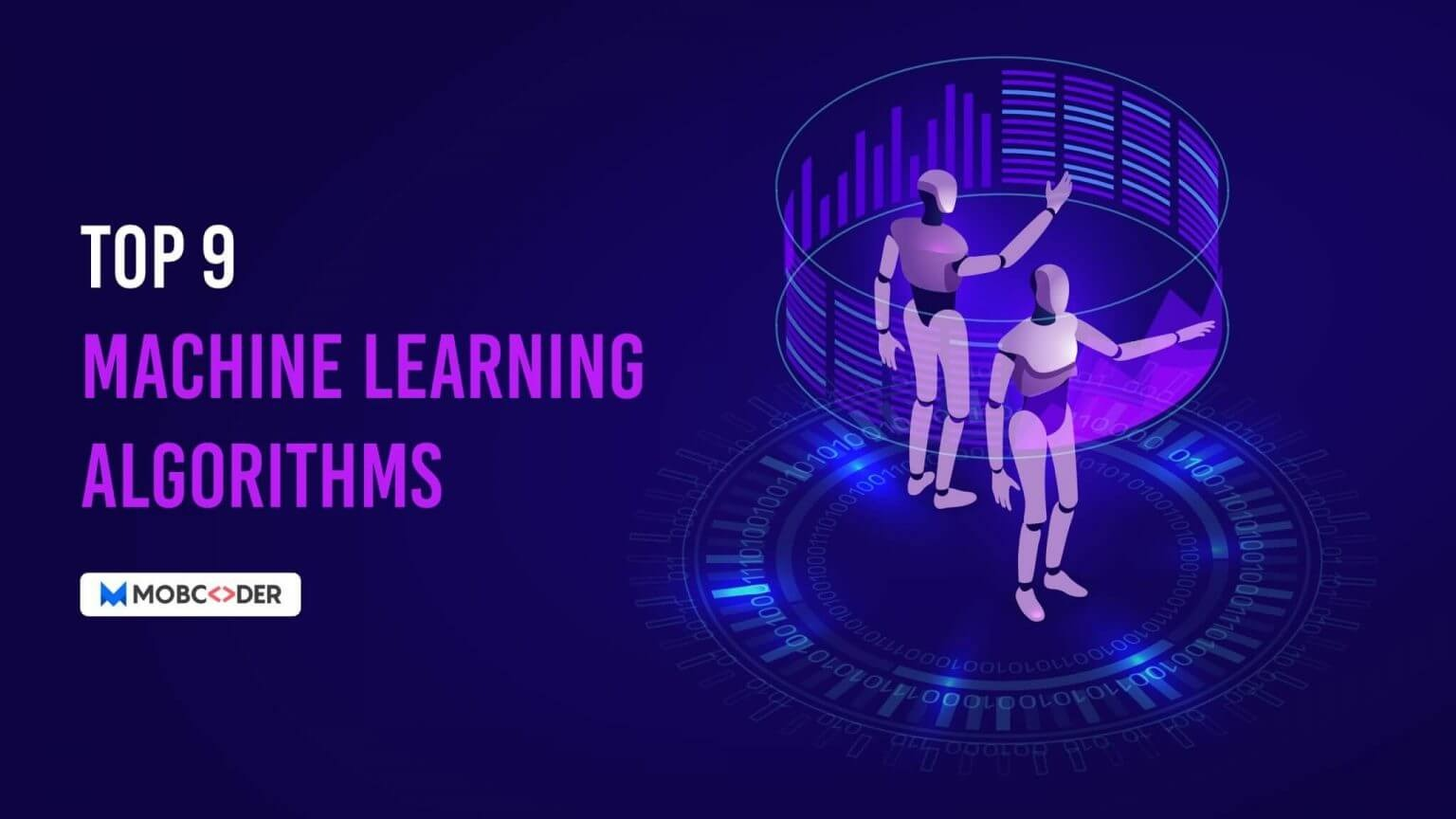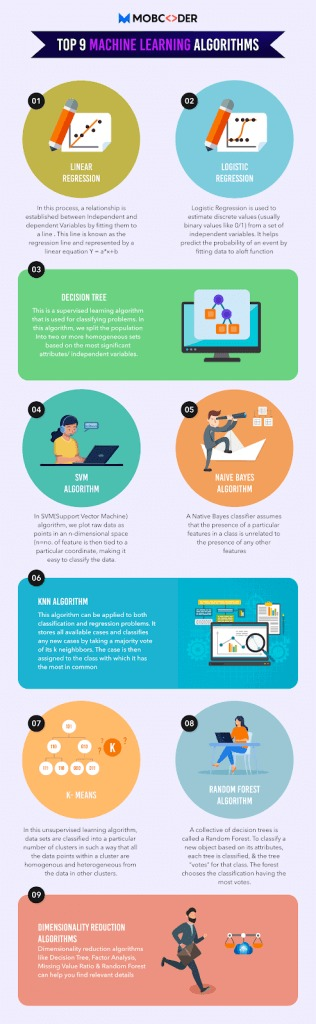# Top 9 Machine Learning Algorithms: Analytics Steps You Need To Know## Introduction:

With nearly maximum manual tasks being automated, It is no doubt that the definition of manual is changing in today’s world. The sub-field of machine learning algorithms has gained much popularity in the tech world at the moment.

Machine learning is incredibly powerful and helps computers predict and calculate more smartly than ever before. Fortunately, we live in an era of technological progress where computing has moved from large mainframes to PCs to the cloud. Before we proceed, it’s time to understand what exactly is ml all about.

## What is ML?

Stands for Machine Learning (ML) is defined as the field of study that automates or improves the capability of computers without being explicitly programmed i.e without any human assistance.

## What is the ML model?

ML model is the model artifact that is developed by the training process and is used to get predictions on new data.

Looking at this, we shall predict what’s next with the ml algorithm in the days ahead.

One of the popular characteristics of machine learning algorithms is the democratization of computing tools and techniques that has enthralled each one of us. During the last few years, data scientists have built sophisticated data-crunching machines with seamless and advanced techniques. The results have been astonishing

Welcome to the world of data science!

### Various Types of Machine Learning Algorithms:

There are broadly 3 types of Machine learning algorithms, you need to understand:

### Supervised Learning –

This is one of the types of machine learning that contains a dependent variable or outcome variable to be predicted from a given set of predictors. This set of variables help generate a function that maps inputs to exact outputs.

This is one of the popular types of machine learning algorithms that makes predictions and is corrected by the operator until it achieves the desired accuracy/performance.

Examples of Supervised Learning: Regression, Decision Tree, Random Forest, etc

### Unsupervised Learning –

Unsupervised learning is one of the types of machine learning algorithms that study data to identify patterns.

This algorithm doesn’t contain any target or outcome variable to be predicted. It is used for clustering populations in several groups that are broadly used for bifurcating customers in different groups for a particular intervention.

Examples of Unsupervised Learning: Apriori algorithm, K-means.

### Reinforcement Learning –

Reinforcement Learning is one of the types of machine learning algorithms that train the machine to make specific decisions. See how this works: the machine trains itself continually using the trial and error method where it learns from past experience.

With the best possible knowledge, the machine then tries to make accurate business decisions.

Example of Reinforcement Learning: Markov Decision Process.

The potential of machine learning to bring value out of data has been adopted majorly by big businesses in many different industries. Most machine learning models are specifically designed and enforced with several algorithms with minor changes.

## Top 9 Machine Learning Algorithms:

Here is a list of the top 9 machine learning methods to identify dangerous cracks in the context of a problem, often a business problem.

1. Linear regression
2. Logistic regression
3. Decision tree
4. SVM algorithm
5. Naive Bayes algorithm
6. KNN algorithm
7. K-means
8. Random forest algorithm
9. Dimensionality reduction algorithms

Let’s understand each ml algorithm in detail:### 1. Linear Regression

To understand how this algorithm functions, let’s take an example from childhood.

Imagine if you are asked to arrange random logs of wood as per the increasing order of their weight.

However – you cannot weigh each log of wood. All you need to do is the visual analysis of the log by looking at its weight and height. Then, use this combination of visible parameters. That’s what linear regression is like.

In this whole process, a new relationship is formed between the two –  independent and dependent variables by fitting them to a line, known as the regression line, and is represented by a linear equation Y= a *X + b, where:

• Y – Dependent Variable
• a – Slope
• X – Independent variable
• b – Intercept

### 2. Logistic Regression

Don’t get confused by its name! It is not a regression algorithm. It is a classification technique that estimates the Binary values like 0/1, true/false, etc depending on the given set of independent variables (s).

It is called Logistic Regression because it predicts the probability of occurrence of an event with the output values lying between 0 and 1 by fitting data to a logit function.

Below methods help improve this type of Logistic algorithm model:

• eliminate features
• regularize techniques
• use a non-linear model

### 3. Decision Tree

The Decision Tree is the most popular ml algorithm in demand today. It falls into the category of a supervised learning algorithm for classifying issues.

This algorithm surprisingly works great for classifying both categorical and continuous dependent variables: where the population is split into two or more homogeneous sets driven from the most significant attributes/ independent variables.

The Decision Tree is one of the machine learning algorithms that is frequently used based on the most significant attributes/ independent variables.

### 4. SVM (Support Vector Machine) Algorithm

SVM algorithm is a classification method. In this machine learning algorithm,  you plot data points in an n-dimensional space (where ‘n’ depicts the number of features you have).

The value of each feature is the value of a particular coordinate, making the classification easier.

For example, suppose you have only two features – Height and Hair length of an individual, you will first plot these two variables in two-dimensional space where each point has two coordinates (also called ‘Support Vectors’)

### 5.  Naive Bayes Algorithm

It is another technique of classification that is based on Bayes’ theorem which assumes the independence between predictors.

In other words, the Naive Bayes algorithm is not a single algorithm but a family of classification algorithms used for discriminating different objects based on specific features. This classifier is used as machine learning modeling for the classification task.

Suppose, let’s consider a fruit – Apple which is red, round, and about 3 inches in diameter. A naive Bayes classifier acknowledges all properties to predict that this fruit is an apple.

This is one of the commonly used machine learning algorithms that are easy to build and mainly used for large data sets.

### 6. KNN (K- Nearest Neighbors) Algorithm

This is one of the top machine learning algorithms that are useful for both classification and regression problems. It’s more broadly used within the Data Science industry for solving classification problems.

All available cases are stored easily along with the classification of cases by taking a majority vote of its k neighbors. Then, this case is then assigned to the class with which it has the most in common. A distance function performs this measurement.

Let’s understand KNN with a real-life example – Suppose you need to find out the information about a person, it makes sense to communicate to his/her friends or colleagues.

### 7. K-Means

It is an unsupervised ml algorithm that resolves clustering problems. In this algorithm, data sets are classified into a particular set of clusters named k.

Hence, data points present within this cluster are both homogeneous and heterogeneous from the data present in other clusters.

### 8. Random Forest Algorithm

Random Forest is a trademarked term for collective decision trees. Each tree is classified and ‘votes’ for that class to classify a new object based on its features.

The forest then selects the classification with maximum votes (over all the trees in the forest).

### 9. Dimensionality Reduction Algorithms

In recent years, a large amount of data are stored and captured in great detail by corporations, government agencies, and research organizations. Some of the machine learning algorithms that can help you find relevant details are Decision Tree, Factor Analysis, Missing Value Ratio, and Random Forest.

Raw data contains a lot of information but the challenge lies in identifying significant patterns and variables. Let’s understand this last but powerful machine learning algorithm with an example.

E-commerce companies are able to fetch maximum details about customers to bring personalized approach than any other nearby shopkeeper. The details are related to their demographics, web crawling history, what they like or dislike, purchase history, reviews, etc.

### Final Note –

By now we are sure that you got the idea of commonly used machine learning algorithms that will sooner provide solutions to complex work challenges.

Identify the problems, develop a broad understanding of the process, apply these algorithms and see the fun!